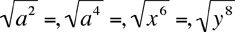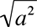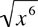Find each square rootHi Diana, Let's do a couple of example with numbers first. √4 = 2 since 22 = 4, in other words 22 = 4 √9 = 3 since 33 = 9, in other words 32 = 9 √64 = 8 since 88 = 64, in other words 82 = 64 So what about? aa = a2 and hence= a. And? x3x3 = x6 and hence= x3 Can you now do the remaining two problems? Harley